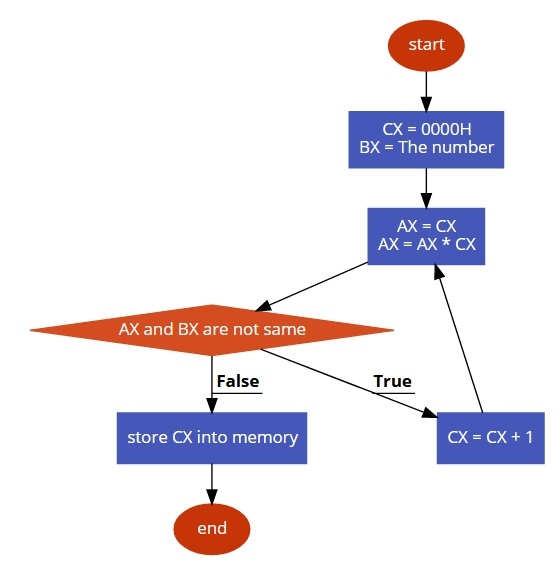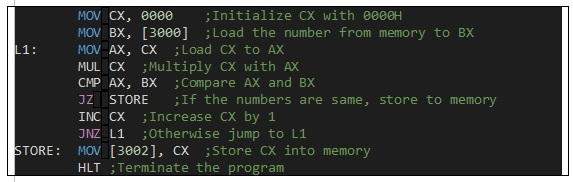# 8086 program to find the square root of a perfect square root number

In this program we will see how to find the square root of a perfect squared.

## Problem Statement

Write 8086 Assembly language program to find the square root of a perfect squared number. The number is stored at memory address 3000. Finally store the result at memory address 3002.

## Discussion

For the perfect square number starting from 0 we are performing square of it, then check whether it is same as the given number or not. If they are same then the current value will be the square root.

For a number 51H (81D), we will check 02, 12, 22, ….. , 92. After 92 it is 51H. So the 9 is square root.

Data

3000
51
3001
00

## Flow Diagram## Program